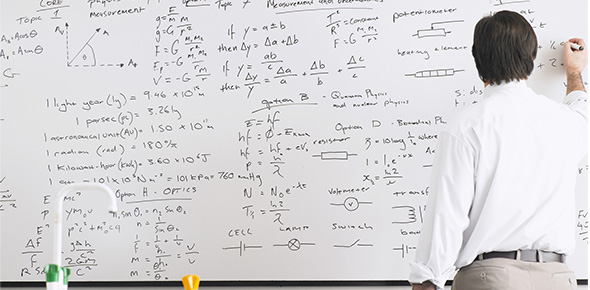Chemical Reactions And Balancing Chemical Equations Under Mr. Tan

10 Questions | Total Attempts: 1002SettingsThis quiz will be your sixth formal assessment. Good luck.

• 1.
Ca(OH)2 + HCl ---> CaCl2+ H2OWhat type of reaction is this?
• A.

Combustion

• B.

Single replacement

• C.

Double displacement

• D.

Combination

• E.

Decomposition

• 2.
C3H8(g) + O2(g) ----> CO2(g) + H2O(g)What type of reaction is this
• A.

Combustion

• B.

Double displacement

• C.

Combination

• D.

Single replacement

• E.

Decomposition

• 3.
C3H8(g) + O2(g) ----> CO2(g) + H2O(g)Balance this reaction. What are the coefficients for each reactant/product in the reaction?
• A.

1,4, 3, 4

• B.

3, 5, 4, 1

• C.

3, 3, 4, 2

• D.

1,3, 5, 2

• E.

1, 5, 3 , 4

• 4.
Ca(OH)2 + HCl ---> CaCl2+ H2OBalance this reaction. What are the coefficients for each reactant/product in the reaction?
• A.

1, 2, 1, 2

• B.

2, 2, 1, 3

• C.

1, 3, 4, 1

• D.

3, 3, 4, 1

• E.

2, 3, 1, 2

• 5.
Cu + HNO3 Cu(NO3)2 + NO + H2OBalance this reaction. What are the coefficients for each reactant/product in the reaction?
• A.

3, 4, 6, 5

• B.

3, 8, 3, 2, 4

• C.

2, 4, 4, 7, 2

• D.

1, 5, 4, 7, 2

• E.

6,2 , 2,4

• 6.
Cu + HNO3 Cu(NO3)2 + NO + H2OWhich of the following is a reactant?
• A.

Cu(NO3)2

• B.

NO

• C.

H2O

• D.

HNO3

• E.

None of these

• 7.
2H20 --> 2H2 + O2What type of chemical reaction is this?
• A.

Combustion

• B.

Combination

• C.

Single displacement

• D.

Double displacement

• E.

Decomposition

• 8.
Al + O2  -->  Al2O3What are the coefficients that would balance this reaction?
• A.

2, 3, 3

• B.

3, 4, 4

• C.

4, 3, 2

• D.

2, 4, 3

• E.

1, 3 ,2

• 9.
C2H6 + O2 --> CO2 + H2OBalance this reaction. What are the coefficients for each reactant/product in the reaction?
• A.

3, 3, 2, 4

• B.

1, 2, 2, 3

• C.

4, 2 , 3 , 4

• D.

2, 7 , 4 ,6

• E.

None of the above

• 10.
Tin (IV) oxide reacts with hydrogen gas to form water and solid tin. This reaction can be represented by
• A.

SnO2(s) → Sn(s) + 2 H2O(g)

• B.

Sn(s) + SnO2(s) → ) 2 H2(g)

• C.

SnO2(s) +H2O(g) → Sn(s) + 22 H2(g)

• D.

SnO2(s) + 2 H2(g) + Sn(s)→ 2 H2O(g)

• E.

2 H2(g) + SnO2(s) + → Sn(s) + 2 H2O(g)

Related TopicsBack to top